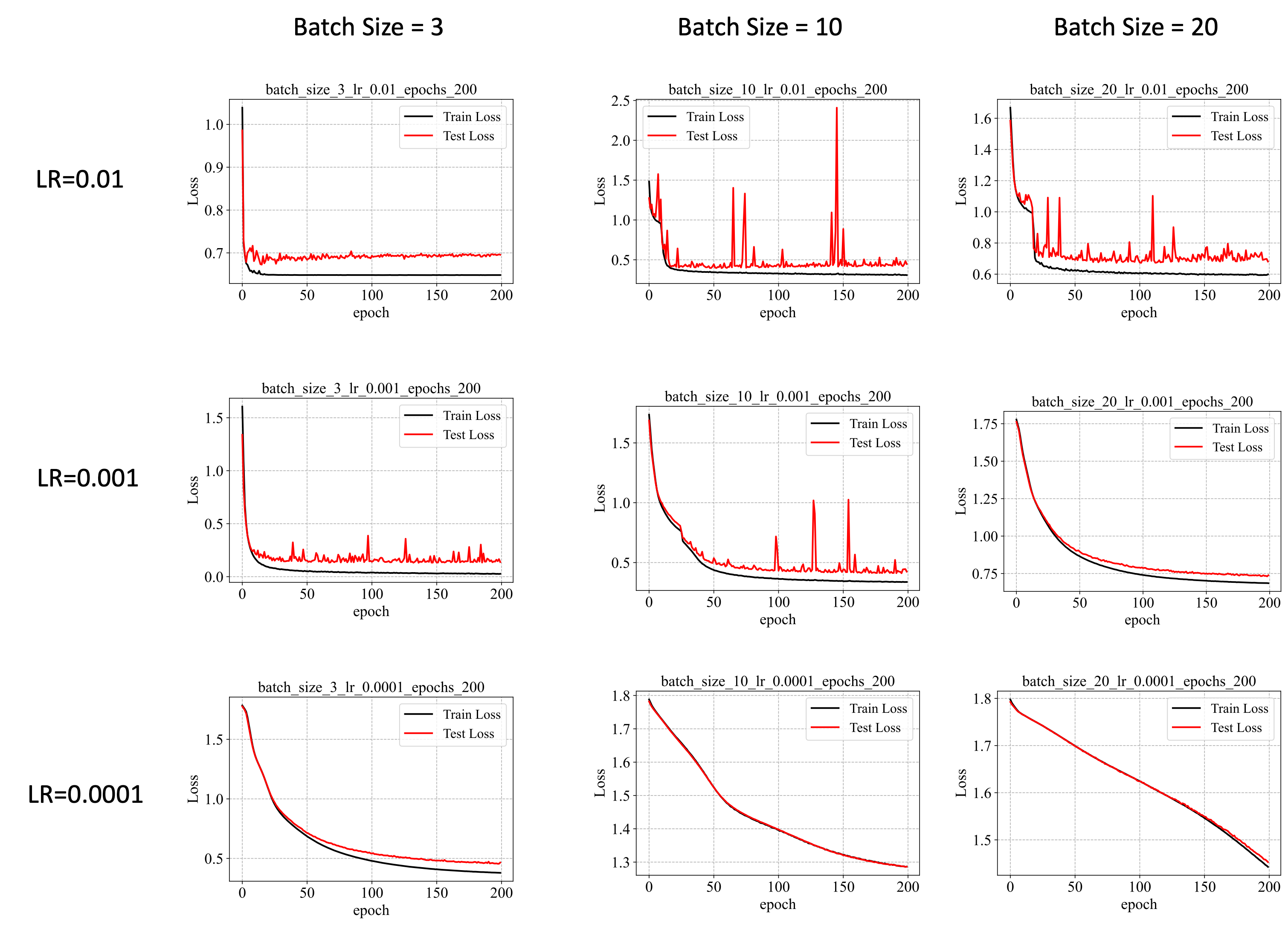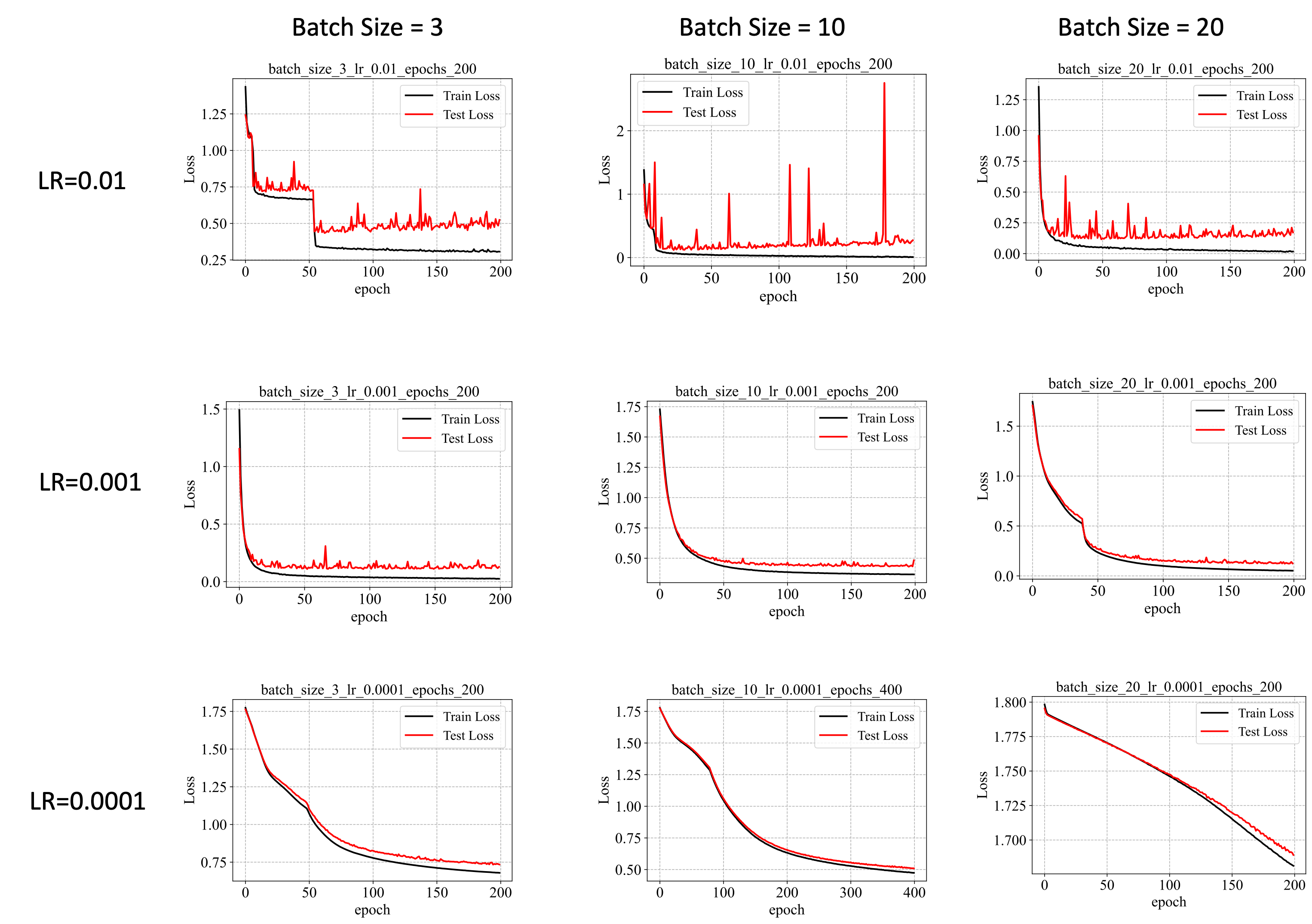# Hyperparameter Tuning on UCI Human Activity Example

This blog shows how to tune the hyperparameters (e.g., batch size, learning rate, # of nodes in each layer) with the UCI Human Activity Example.

The code can be found here.

The model is a simple three-layer NN model, and the hidden layer has 100 nodes.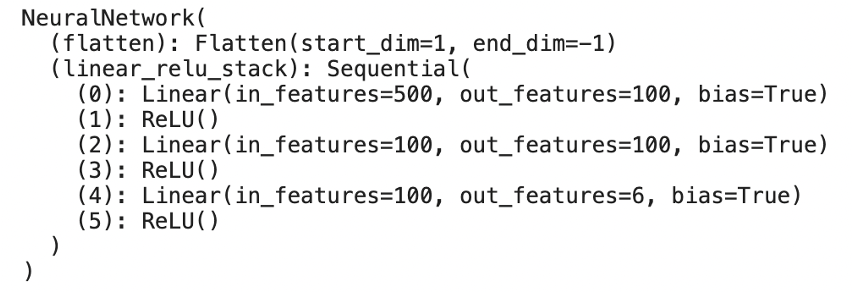I tried different combination of batch size (3, 10, 20) and learning rate (1e-2, 1e-3, 1e-4). The results are shown in the following.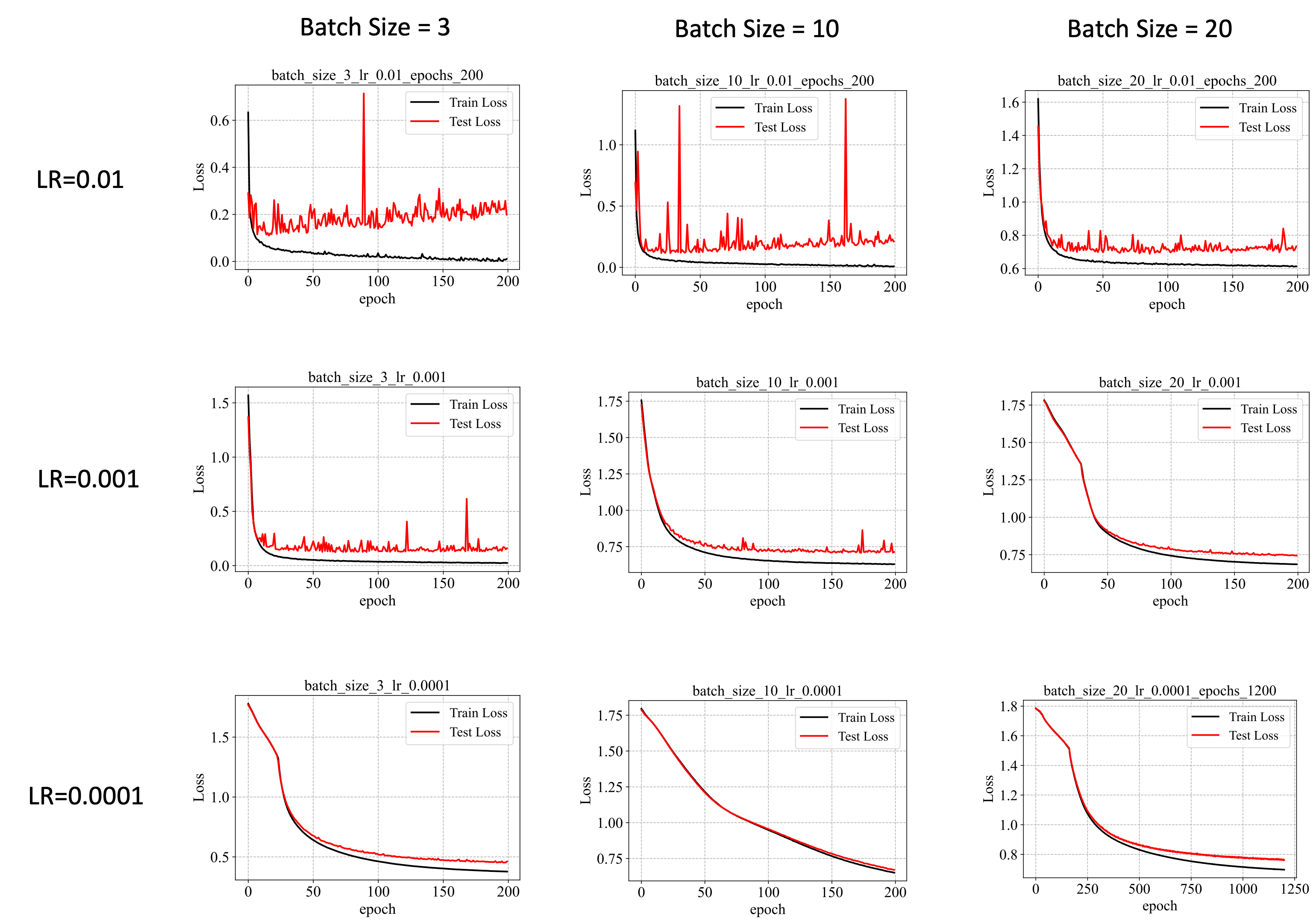Based on the above results, we have the following observations:

• A large learning rate leads to a faster learning process and larger variations in loss values in the test set.
• A smaller batch size leads to a faster learning process.
• A large learning rate does not necessarily lead to smaller test loss.

The best performance achieved (test loss is around 0.2) is shown in the following.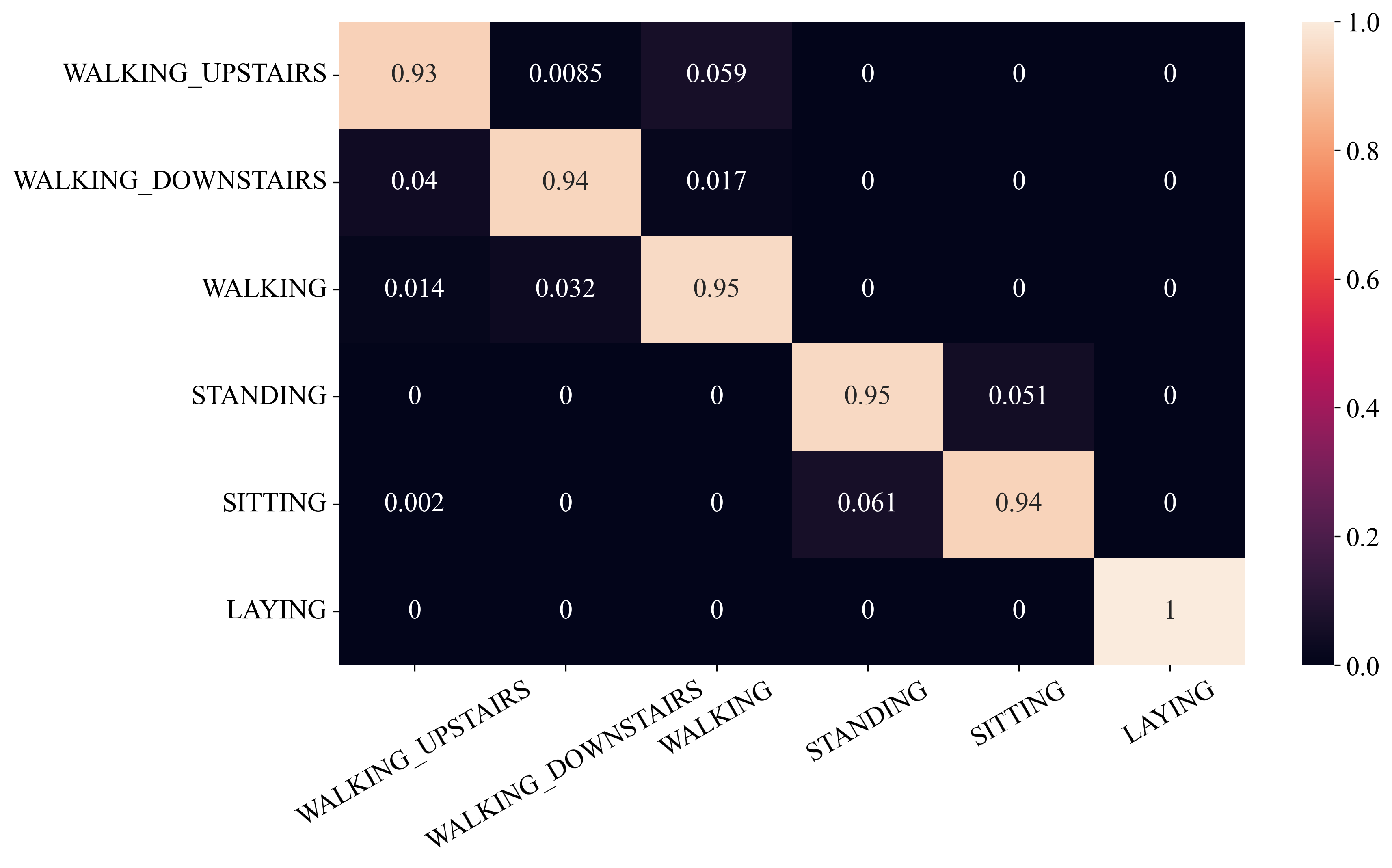Similar results can be observed when we set the hidden layer size to 50 and 200.